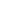# The sum of the rwo digits of two digit number is 11 and the difference of the first digit from the second is 5. The two digit number is

a) 38
b) 83
c) 74
d) 27
KPSC Divisional Accountant-15

 38. Solution Let 'ab' be the two digit number. a+b = 11 b-a = 5 b = 8, a = 3
Share :
+
Next
« Prev
Prev
Next »